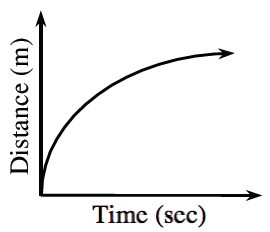### Home > APCALC > Chapter 3 > Lesson 3.3.1 > Problem3-90

3-90.

The graph below shows the distance from a fixed point traveled by a toy car. Use the graph to sketch the velocity of the car.

Is the velocity graph going to have positive $y$-values, negative $y$-values or both?

As $x$ increases, does the velocity increase or decrease?

Does the velocity ever reach $0$?